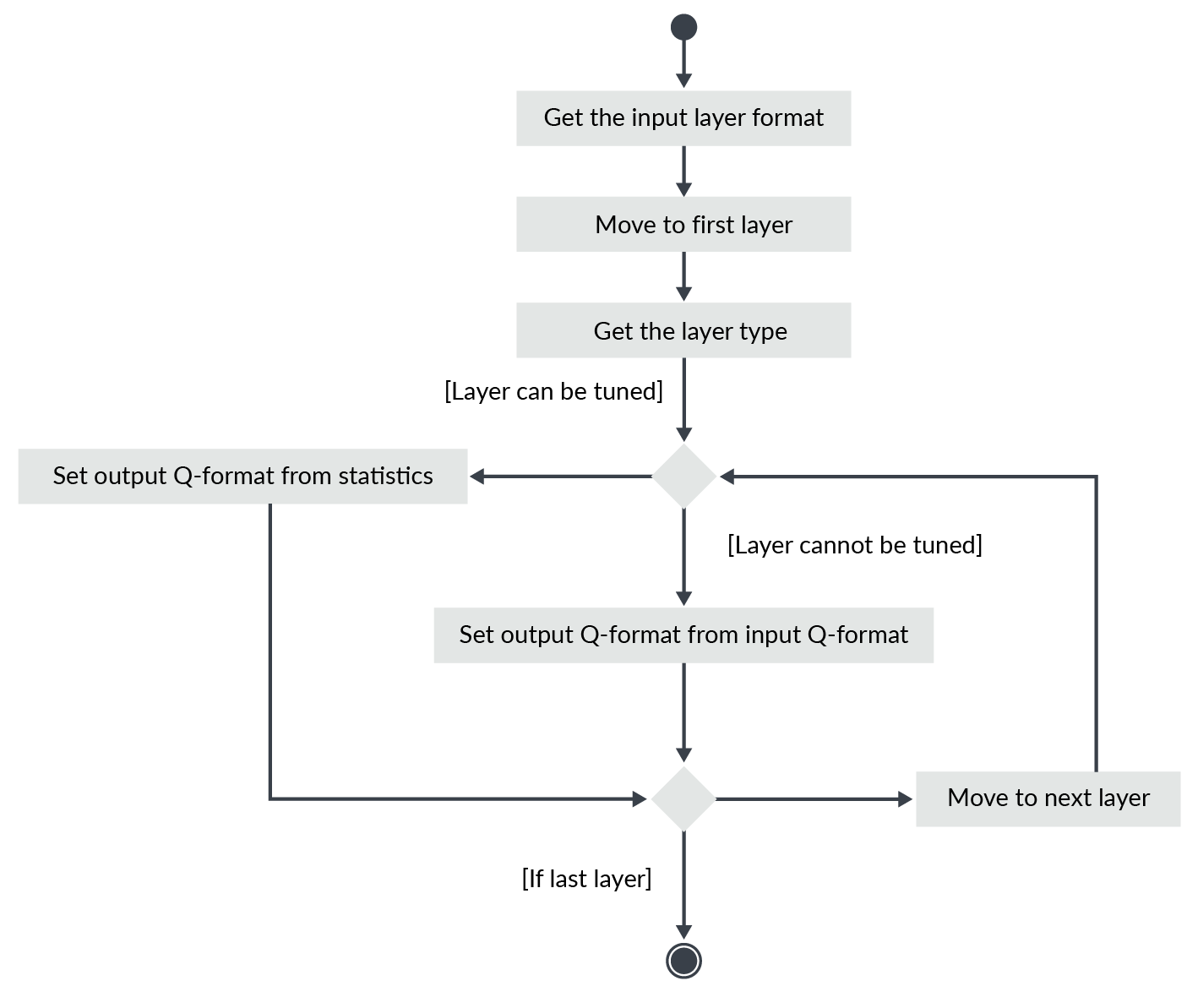## Compute the layer Q-formats

Once you have statistics for each layer and a choice of a quantization scheme, you can deduce the Q-format for the inputs and outputs of the layers.

The Q-formats computed from the statistics and word size are based upon the assumption that the output format can be chosen independently from the input one. It is not possible for all layers.

Some layers impose constraints on the output format. For instance, the Q-format of the output of a max pool is the same as its input format, because of how the algorithm is implemented in CMSIS-NN.

Therefore, you cannot freely choose the output format for a max pool layer even if, according to the statistics, a better choice may be possible.

It is only for fully connected layers and convolutional layers that it is possible to choose the output format independently from the input format by shifting the biases and the output values.

At this step you need to choose a Q-format for each input and output. You need to consider:

• How the layers are connected
• Which layers are allowing to customize the output format

Start with the network input Q-format based on the statistics of the training patterns. If the input layer is a fully connected or convolutional one, then define the output Q-format based upon the output statistics. Otherwise, the output Q-format is computed from the input format and the nature of the layer.

Iterate the procedure one layer after another. At the end you should have a list of Q-format for all the input and output of the layers.

This procedure is summarized in the following diagram: